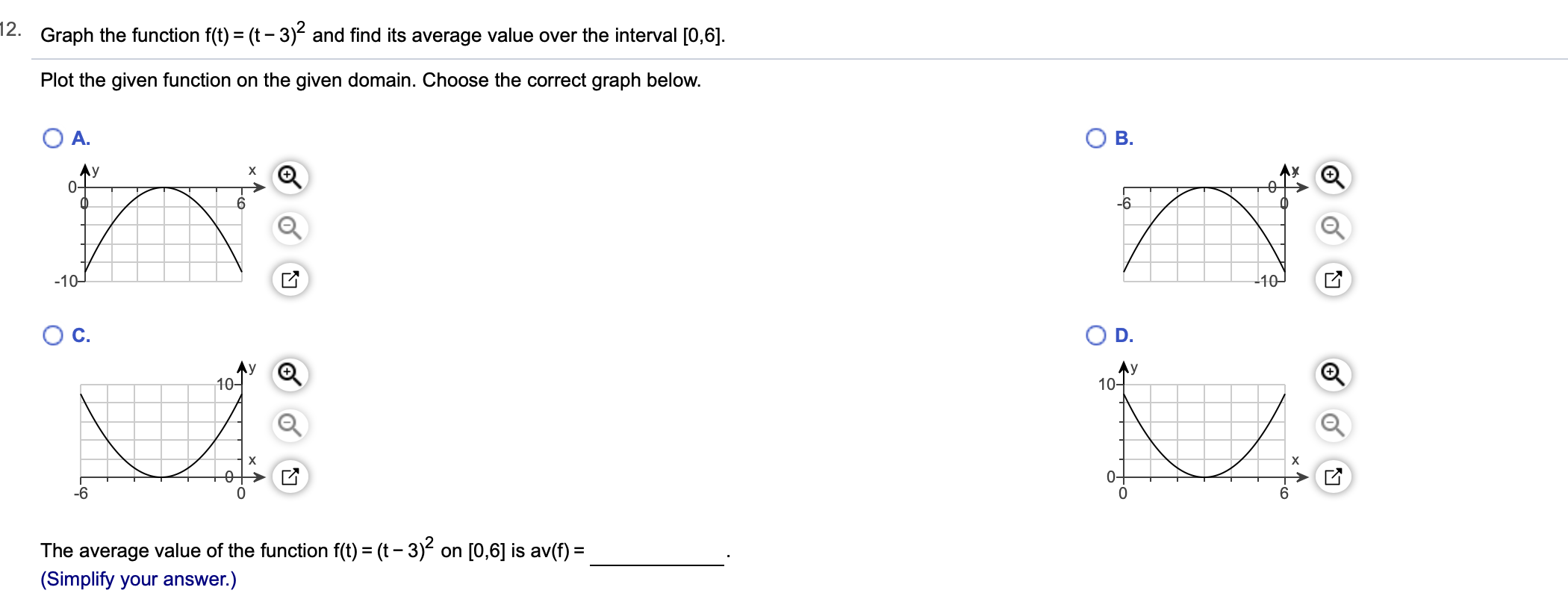# 12.Graph the function f(t) (t-3) and find its average value over the interval [0,6]Plot the given function on the given domain. Choose the correct graph below.A.В.AyAxX-6-10-10Ос.ЛУ О10-Ay10X040-6The average value of the function f(t) (t-3) on [0,6] is av(f)(Simplify your answer.)

Question
4 views

Can you help with this problem step by step?help_outlineImage Transcriptionclose12. Graph the function f(t) (t-3) and find its average value over the interval [0,6] Plot the given function on the given domain. Choose the correct graph below. A. В. Ay Ax X -6 -10 -10 Ос. ЛУ О 10- Ay 10 X 04 0 -6 The average value of the function f(t) (t-3) on [0,6] is av(f) (Simplify your answer.) fullscreen
check_circle

Step 1

Given,

Step 2

It is in the form of y = x2   and so it is an upward parabola with ver...

### Want to see the full answer?

See Solution

#### Want to see this answer and more?

Solutions are written by subject experts who are available 24/7. Questions are typically answered within 1 hour.*

See Solution
*Response times may vary by subject and question.
Tagged in

### Calculus